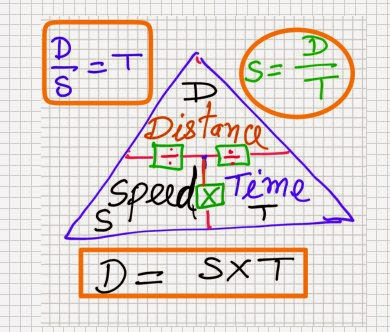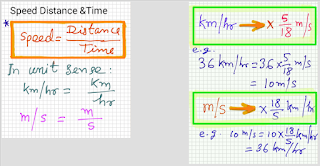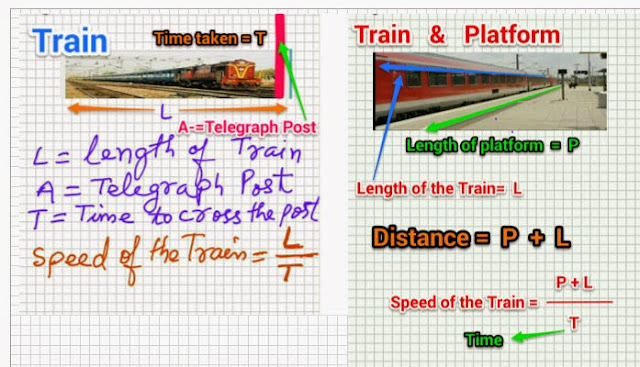Mar 17, 2015

# Speed Distance Time Math Class 8 Part 1The most important thing about this chapter is that the sums are very easy and can be solved by most of the students if the formula is known to him. Also it is more important to keep in memory the formula of speed, distance and time. The third most important point to note is the unit of speed given in the sum and units of distance and time. It is here the student's minds are confused. Be clear about km/hr and m/sec and mile/hr.

For converting km/hr to m/s multiply by as it can be noticed in the above image. And for converting m/s to km/hr multiply by 18/5Sometime it is written as :
60 kmph = it means 60 km/hr
60 mph = it means 60 m/hr or 60 mile/hr

# You may also Like These !